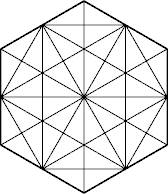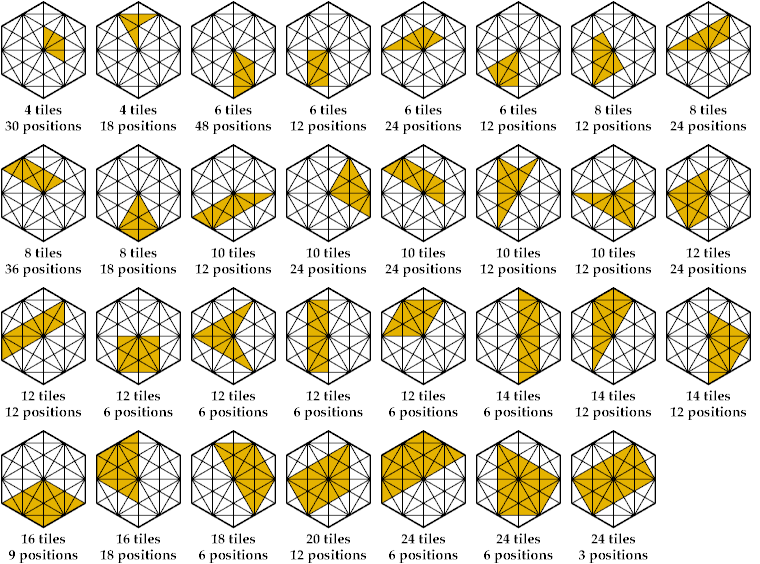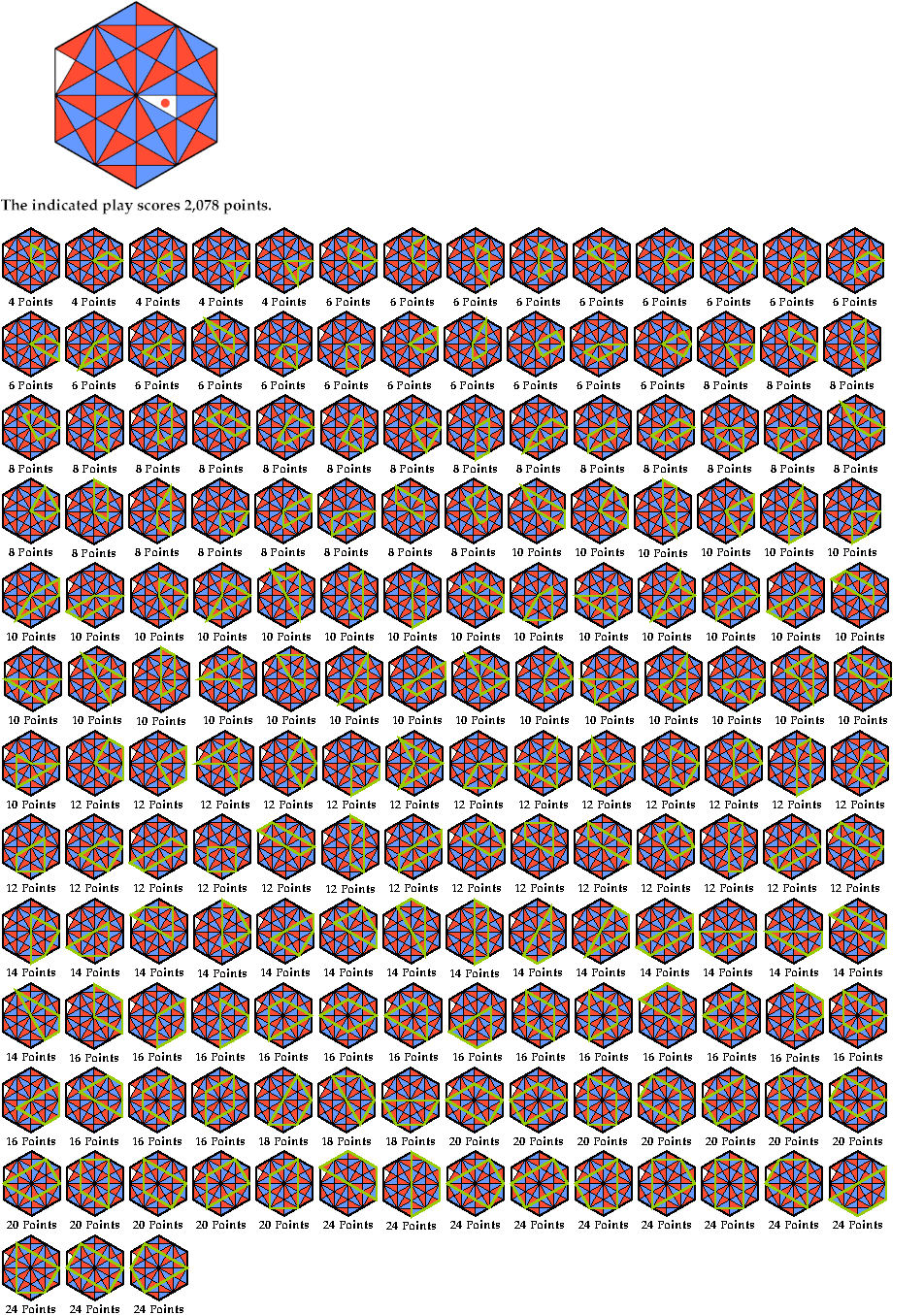# The Highest-Scoring Play in Quadrigon

Wilson Ransome's game of Quadrigon appeared in 1976 in Games and Puzzles. It is played on a hexagonal board divided into 48 right triangles:Two or three play. Each chooses a different color. The players then take turns placing a triangle of their color into a vacant space. After the first turn, a new triangle must touch at least one previous triangle at at least one point. The game ends when all spaces are occupied.

Each play that completes a quadrilateral on the board scores as many points as the number of triangles in the quadrilateral, subject to these conditions:

• The quadrilateral must be fully occupied.
• The quadrilateral must have more than 2 triangles.
• Exactly half the triangles in the quadrilateral must belong to the current player.
• The outline of the quadrilateral may not pass between two adjacent triangles of the same color.

Because of the third condition, a quadrilateral can be scored only if it contains an even number of triangles. Here is a picture of the 31 sizes and shapes of scorable quadrilaterals on the board.The board contains 468 such quadrilaterals.

The highest possible score on a single play in Quadrigon is 2,078 points. Here is the scoring:Thanks to Jonathan Clark for giving me a Quadrigon set—in fact, two of them.

Last revised 2015-10-01.

Col. George Sicherman [ HOME | MAIL ]